Chapter 7 Consumer choice Utility n n Utility

• Slides: 22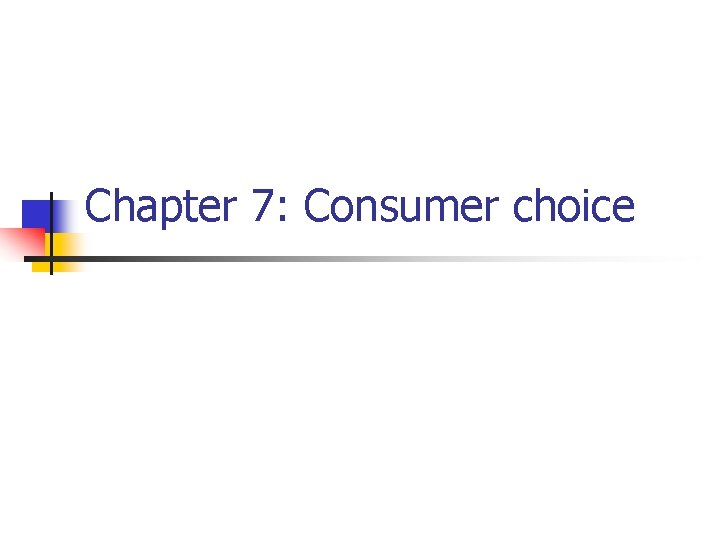Chapter 7: Consumer choice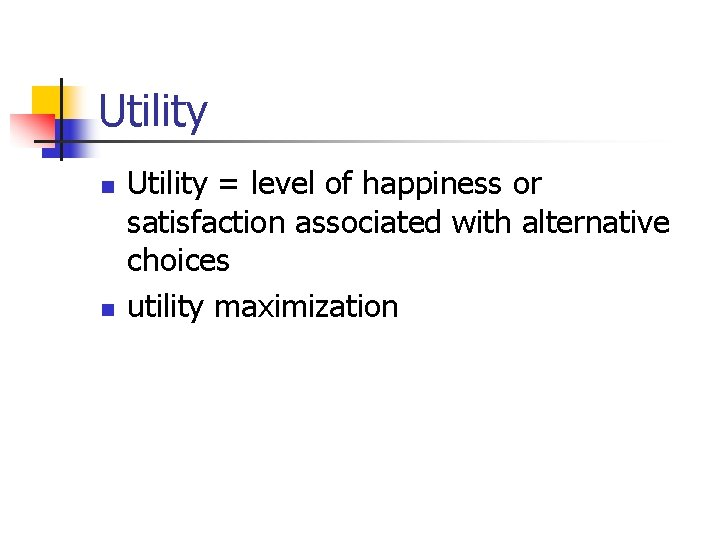Utility n n Utility = level of happiness or satisfaction associated with alternative choices utility maximization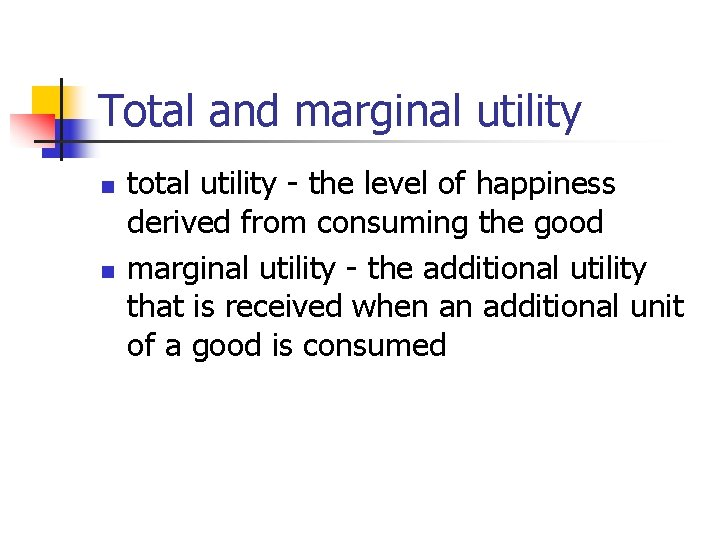Total and marginal utility n n total utility - the level of happiness derived from consuming the good marginal utility - the additional utility that is received when an additional unit of a good is consumed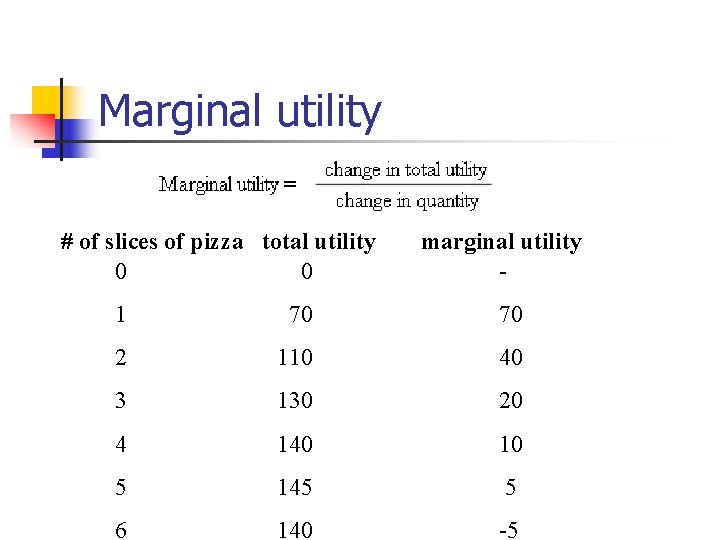Marginal utility # of slices of pizza total utility 0 0 marginal utility - 1 70 70 2 110 40 3 130 20 4 140 10 5 145 5 6 140 -5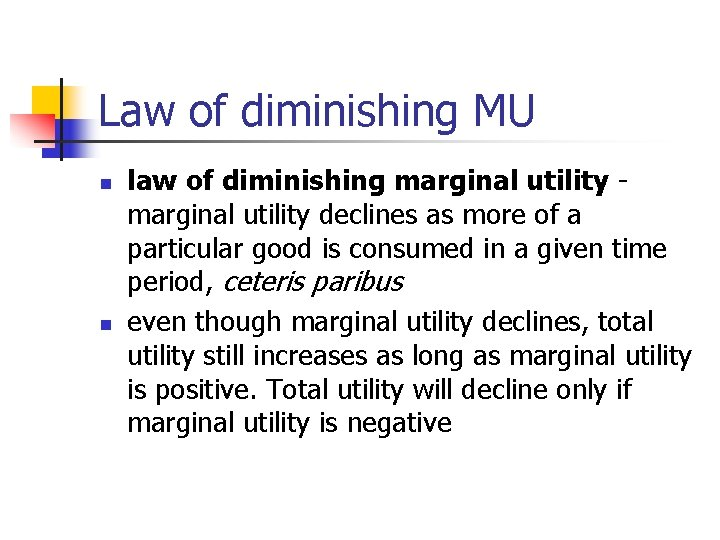Law of diminishing MU n n law of diminishing marginal utility declines as more of a particular good is consumed in a given time period, ceteris paribus even though marginal utility declines, total utility still increases as long as marginal utility is positive. Total utility will decline only if marginal utility is negativeDiamond-water paradox n n n As noted by Adam Smith, water is essential for life and has a low market price (often a price of zero) while diamonds are not as essential yet have a very high market price. Smith’s explanation: “value in use” vs. “value in exchange” value in exchange: labor theory of valueMU of water and diamonds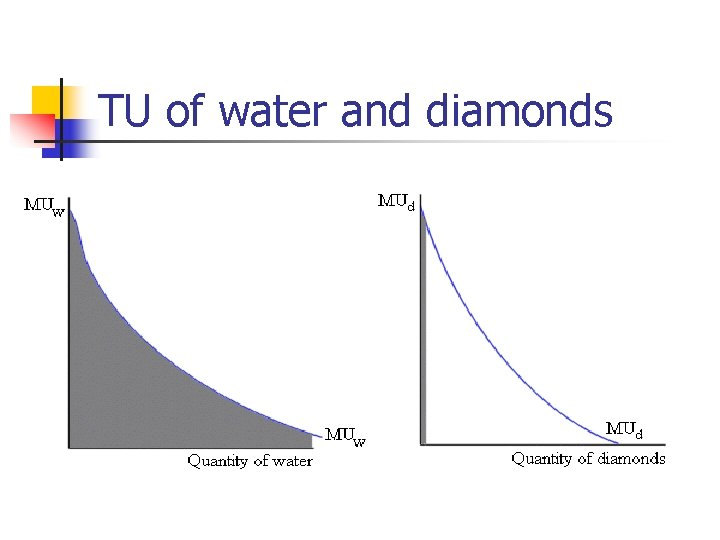TU of water and diamondsValue in use and value in exchange n n value in use = total utility value in exchange is related to marginal utilityConsumer equilibrium 1. 2. All income is spent. The first condition listed above is sometimes referred to as the "equimarginal principle. "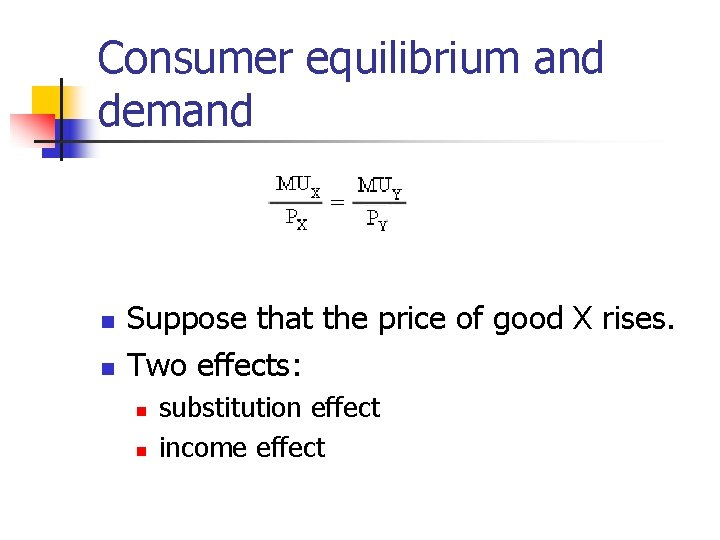Consumer equilibrium and demand n n Suppose that the price of good X rises. Two effects: n n substitution effect income effect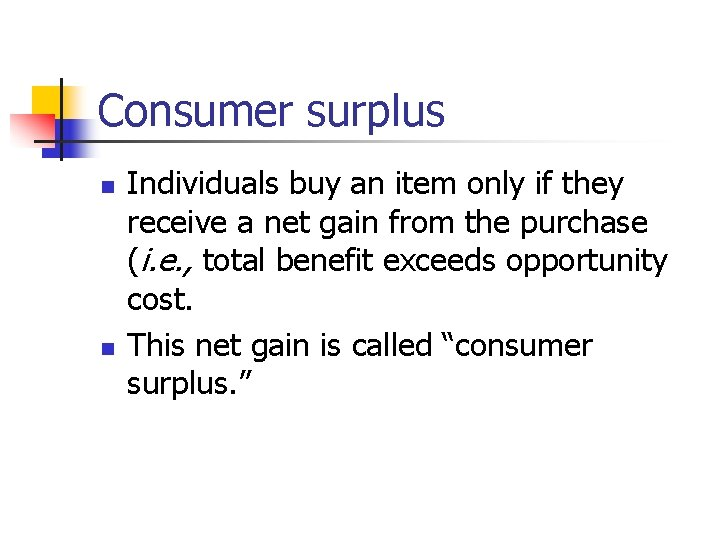Consumer surplus n n Individuals buy an item only if they receive a net gain from the purchase (i. e. , total benefit exceeds opportunity cost. This net gain is called “consumer surplus. ”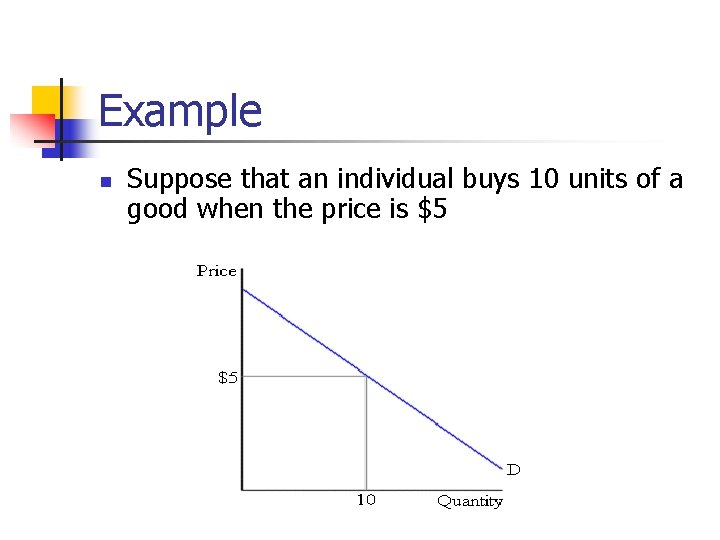Example n Suppose that an individual buys 10 units of a good when the price is \$5Benefits and cost of first unit • Benefit = blue + green rectangles (=\$9) • Cost = green rectangle (=\$5) • Consumer surplus = blue rectangle (=\$4)Total benefit to consumerTotal cost to consumer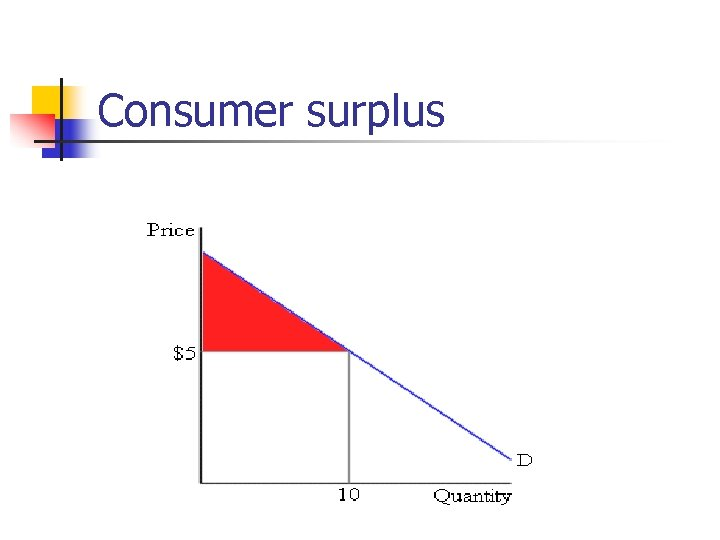Consumer surplusIndifference curves n n Indifference curve – a graph of all of the combinations of goods that provide a given level of utility Any two points on an indifference curve provide the same level of utilityPoints on and off an indifference curve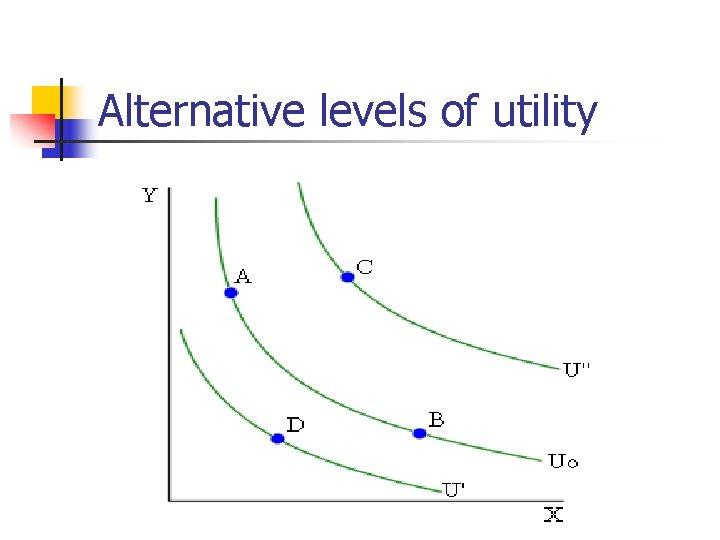Alternative levels of utilityBudget constraintOptimum consumption bundle